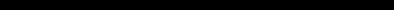# SINCLAIR ZX SPECTRUM BASIC ProgrammingCHAPTER 12
Arrays

#### Summary

Arrays (the way the ZX Spectrum handles string arrays is slightly non-standard).

DIM ...

Suppose you have a list of numbers, for instance the marks of ten people in a class. To store them in the computer you could set up a single variable for each person. but you would find them very awkward. You might decide to call the variable Bloggs 1, Bloggs 2, and so on up to Bloggs 10, but the program to set up these ten numbers would be rather long and boring to type in.

How much nicer it would be if you could type this:

5 REM this program will not work
10 FOR n=1 TO 10
30 NEXT n
40 DATA 10,2,5,19,16,3,11,1,0,6

Well you can't.

However, there is a mechanism by which you can apply this idea, and it uses arrays. An array is a set of variables, its elements, all with the same name, and distinguished only by a number (the subscript) written in brackets after the name. In our example the name could be b (like control variables of FOR - NEXT loops, the name of an array must be a single letter), and the ten variables would then be b(l), b(2), and so on up to b(l0).

The elements of an array are called subscripted variables, as opposed to the simple variables that you are already familiar with.

Before you can use an array, you must reserve some space for it inside the computer, and you do this using a DIM (for dimension) statement.

DIM b(10)

sets up an array called b with dimension 10 (i.e. there are 10 subscripted variables b(1),...,b(10)) and initializes the 10 values to 0. It also deletes any array called b that existed previously. (But not a simple variable. An array and a simple numerical variable with the same name can coexist, and there shouldn't be any confusion between them because the array variable always has a subscript).

The subscript can be an arbitrary numerical expression, so now you can write

10 FOR n=1 TO 10
30 NEXT n
40 DATA 10,2,5,19,16,3,11,1,0,6

You can also set up arrays with more than one dimension. In a two dimensional array you need two numbers to specify one of the elements - rather like the line and column numbers to specify a character position on the television screen - so it has the form of a table. Alternatively, if you imagine the line and column numbers (two dimensions) as referring to a printed page, you could have an extra dimension for the page numbers. Of course, we are talking about numeric arrays; so the elements would not be printed characters as in a book, but numbers. Think of the elements of a three-dimensional array v as being specified by v (page number, line number, column number).

For example, to set up a two-dimensional array c with dimensions 3 and 6, you use a DIM statement

DIM c(3,6)

This then gives you 3*6=18 subscripted variables

c(1 ,1),c(1 ,2), . . .,c(1,6)
c(2, 1 ),c(2,2), . . .,c(2,6)
c(3, 1 ),c(3,2), . . .,c(3,6)

The same principle works for any number of dimensions.

Although you can have a number and an array with the same name, you cannot have two arrays with the same name, even if they have different numbers of dimensions.

There are also string arrays. The strings in an array differ from simple strings in that they are of fixed length and assignment to them is always Procrustean - chopped off or padded with spaces. Another way of thinking of them is as arrays (with one extra dimension) of single characters. The name of a string array is a single letter followed by \$, and a string array and a simple string variable cannot have the same name (unlike the case for numbers).

Suppose then, that you want an array a\$ of five strings. You must decide how long these strings are to be - let us suppose that 10 characters each is long enough. You then say

DIM a\$(5,10)      (type this in)

This sets up a 5*10 array of characters, but you can also think of each row as being a string:

a\$(1)=a\$(1,1) a\$(1,2) ... a\$(1,10)
a\$(2)=a\$(2,1) a\$(2,2) ... a\$(2,10)
:          :          :               :
a\$(5)=a\$(5,1) a\$(5,2) ... a\$(5,10)

If you give the same number of subscripts (two in this case) as there were dimensions in the DIM statement, then you get a single character; but if you miss the last one out, then you get a fixed length string. So, for instance, A\$(2,7) is the 7th character in the string A\$(2); using the slicing notation, we could also write this as A\$(2)(7). Now type

LET a\$(2)="1234567890"

and
PRINT a\$(2),a\$(2,7)

For the last subscript (the one you can miss out), you can also have a slicer, so that for instance

a\$(2,4 TO 8)=a\$(2)(4 TO 8)="45678"

Remember:

In a string array, all the strings have the same, fixed length. The DIM statement has an extra number (the last one) to specify this length. When you write down a subscripted variable for a string array, you can put in an extra number, or a slicer, to correspond with the extra number in the DIM statement. You can have string arrays with no dimensions. Type

DIM a\$(10)

and you will find that a\$ behaves just like a string variable, except that it always has length 10, and assignment to it is always procrustean.

#### Exercises

 1. Use READ and DATA statements to set up an array m\$ of twelve strings in which m\$(n) is the name of the nth month. (Hint: the DIM statement will be DIM m\$(12,9). Test it by printing out all the m\$(n) (use a loop). Type PRINT "now is the month of ";m\$(5);"ing";" when merry lads are playing" What can you do about all those spaces?